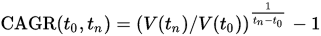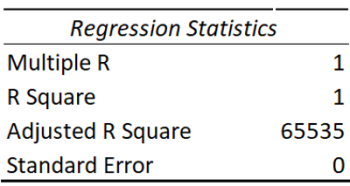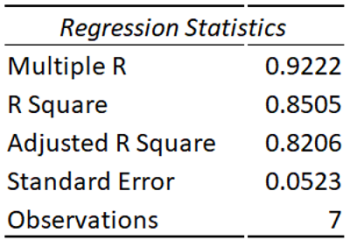# Kingmaker’s State of States 2020 – How They Ranked

The overall State of States ranking from Kingmakers shows how each of the 36 Nigerian states, plus the Federal Capital Territory Abuja, ranked in 47 indicators across five categories.

In calculating the rankings, each of the five categories were assigned equal weightings:

The elements of the categories and the weightings assigned to each were:

#### Living Standards

Note: Weights don’t always add to 100 due to rounding.

Kingmakers created an index score for each metric for each state. In each metric, the top state based on the raw data was given 1 point and the bottom state was given 37 points. States between these were indexed proportionally. For the overall rankings, Kingmakers created an average of the seven category rankings, and then ranked the outcome. We chose this method for the overall ranking so that it would not be skewed by large differences in scores at a metric level.

### The Data

In order to select metrics for the project, we consulted experts in each of the categories for guidance. These are the criteria we used in choosing metrics:
• Metrics that measure citizen outcomes in a state were favoured over inputs or outputs. For example, we selected the percentage of state residents who had finished primary school education or higher instead of the amount spent on education per capita or the number of students enrolled at state schools.
• The set of metrics within each category should work together to provide an overview of that category.
• Standardized data needed to be available across all or most states.
The data behind the rankings were sourced from

### Kingmakers.com Calculations

#### Population

The data for the population for the states was acquired from the National Population Commission and National Bureau of Statistics. We got the total population for each of the states of the years 2006, 2008, 2010 and 2011. We then calculated the compound average growth rate (CAGR) from 2006 to 2011 and used that to extrapolate the population count for the years 2012 to 2015.V(t0): start value, V(tn): finish value, tn – t0: number of years.

The same method was used for calculating for the values for the male and female population as well as the distribution across age groups.

There are a few limitations to using CAGR to forecast metrics and they include:

1. CAGR calculates the smooth average of growth over a period, it ignores volatility and implies that the growth during that time was steady. Yet, this is never actually the case. As such, we cannot take CAGR at face value.
2. CAGR is mainly a historic measurement and , no matter how steady the growth of a metric has been over a period of time we cannot safely assume that the growth will continue at the same rate during the following year or years, as other factors may come into play and affect that rate of growth.
3. Lastly, CAGR has a problem with representation. Say for instance a metric’s CAGR could be an impressive 50% over the past three years. However, due to poor or negative growth in the two years preceding those three years, the CAGR over the past five years could come down to more modest 2.73%.
However, still we adopt a culture of collecting and collating data more periodically, CAGR is one of the tools are our disposal to make calculated guesses to fill in the void for the years no data was collected.

Looking the national data for infant mortality rates and the life expectancy at birth since 1980, we noticed that was a negative correlation between the two metrics. As infant mortality rates drop, life expectancy at birth increased. The data was used to develop a linear regression equation which had the following statisticsThe infant mortality rates were calculated using data from the DHS Program and the applied to the linear regression equation to get the adult life expectancy.

Life Expectancy = 59.87237 – ( 0.111 x 'Infant Mortality Rate' )

#### Maternal Mortality

We also took a look at maternal rates mortality for Nigeria from the DHS Program, which were only available on a national level and found a direct correlation between maternal mortality rates and both the level of antenatal care received and proportion of women experiencing problems with accessing healthcare. A linear regression model was developed using the statistics below:The linear regression equation below was used to project the maternal maternity rates using the state level antenatal care received and proportion of women experiencing problems with accessing healthcare data from the DHS Program.

Maternal Mortality Rates = 1397.51 - ('Antenatal Care Rates' x 10.86) - ('Problem with Access to Care Rates' x 3.06)

#### GDP

The National Bureau of Statistics in 2012 released the Gross Domestic Product (GDP) estimates for each of the states in Nigeria and the Federal Capital Territory, Abuja for the year 2010. This so far has been the only time that the subnational GDP reports have been released.

After going through several models in order to forecast the GDP for each of the state, including the one we used in 2016, we settled on a multiple regression model that forecasted the change in the GDP of each of the state based on the change in the US dollar equivalent of the internally generated revenue (IGR) by the state governments..

We got the data for the IGR for each of the state from the period of 2010 to 2018 from the National Bureau of Statistics. We then converted the amounts we got using the average exchange rate to the US dollar for the year in which the revenue was generated using data from the Central Bank of Nigeria. The annual rate changes for the total revenue generated by the states was then calculated and then compared the change in the GDP of Nigeria and developed the regression model with the following statistics:The regression formula for the annual change in GDP for each of the state is shown below.

GDP Growth Rate =-0.045453743 + (0.772468041 x 'IGR Growth Rate')

Dr. Obi Igbokwe
Founder